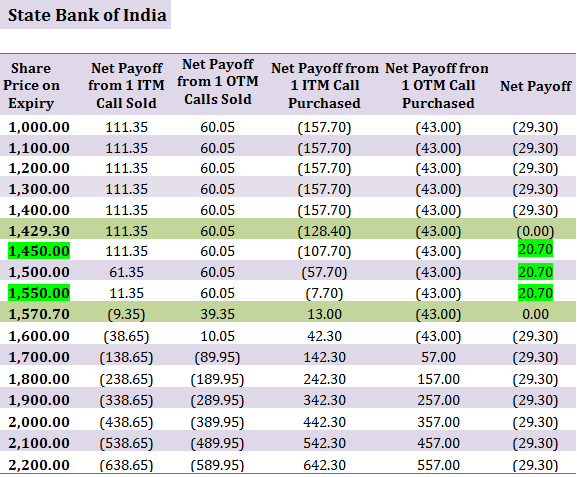When to useLong call condor option strategy is similar to a long call butterfly spread strategy. It is used when the investor believes that the stock is going to trade in a range and will be volatile in the near future.

How it works: Long call condor option strategy uses four option contracts of the same underlying stock with the same expiry date but with different strike prices. In this strategy, you sell/write 1 in-the-money call options  and 1 out-of-the-money call option; buy 1 in-the-money call option and 1 out-of-the-money call option, each with the same expiry date, T.

For example: On 19th August 2013, when the share of State Bank of India was trading at Rs. 1,530.40, you decide to sell/write 1 in-the-money call option at a premium of Rs. 111.35 with a strike price of Rs. 1,450; sell/write 1 out-of-the-money call option at a premium of Rs. 60.05 with a strike price of Rs. 1,550; buy 1 in-the-money call option at a premium of Rs. 157.70, with a strike price of Rs. 1,400; buy 1 out-of-the-money call option at a premium of Rs. 43.00, with a strike price of Rs. 1,600.

(click to enlarge)For Short Call Options: If the price of the share stays below Rs. 1,450 and/or Rs. 1,550 (i.e. respective strike prices of the 2 short call options) until expiry, you will retain partial or the entire premium amount (depending upon where the underlying stock price settles). If however the price rises above Rs. 1,450 and/or Rs. 1,550, the buyer of the call option may exercise his option and make a profit based on how far above does the stock price rise. From the perspective of the seller of the call (you), you will start suffering a loss once the stock price rises above Rs. 1,621.40 (i.e. lower strike price + total premium received on the two short call options).

For Long Call Options: If the price of State Bank of India share rises above Rs. 1,400 and/or Rs. 1,600 (i.e. respective strike prices for the 2 long call options), you can exercise your respective options, but the price of the stock must rise above Rs. 1,600.70 (i.e. lower strike price + total premium paid on the two long call options).

Risk/Reward: In long call condor option strategy, the risk is limited and calculated by subtracting the net premium paid, from the difference between the higher and the lower strike price and  the maximum reward/profit is also limited and will be realized when the stock is trading between the two middle strike prices.

The table below shows the net payoff of long call condor strategy assuming different spot prices on the expiry date:The table above allows you to easily see the break-even points, maximum profit and the loss potential on expiry in rupee terms.

The two break-even points occur when the price of the underlying share equals Rs. 1,429.30 and Rs. 1,570.70.

First Break-even Point = Lowest Strike price + net premium paid = Rs. 1,429.30 (1,400 + 29.30)

Second Break-even Point = Highest Strike price – net premium paid = Rs. 1,570.70 (1,600 – 29.30)

The maximum profit (i.e. Rs. 20.70) will be made if the share price (upon expiry) is anywhere between the two middle strike prices i.e. Rs. 1,450 and Rs. 1,550.

The maximum loss which the investor may suffer is equal to the net premium paid (i.e. Rs. 29.30). Maximum loss, in this example, will be incurred if the share price falls below Rs. 1,429.30 or rises above Rs. 1,570.70 upon expiry.

## How to use the Long Call Condor Option Strategy Excel calculator

Just enter your expected spot price on expiry, option strike price and the amount of premium, to estimate your net pay-off from the Strategy.

Note: The example and calculations are based assuming a single share though in reality options are based on lots of many shares. For example State Bank of India call option contract is for 125 shares. Accordingly the net premium paid will be Rs. 3,662.50 for 4 lots (i.e. 29.30*125) in our example.

Also Note: Unlike the buyer of an option who only pays the premium to buy the option, the seller of an option must deposit a margin amount with the exchange. This is because he takes an unlimited risk as the stock price may rise to any level. In case the price rises sharply above the strike price, the exchange utilises the margin amount to make good the profit which the option buyer makes. The amount of margin is decided by the exchange and it typically ranges from 15 % 60 % based on the volatility in the underlying stock and market conditions. In the above example, as a seller of call option, you will have to deposit a margin of Rs. 46,875.00 (i.e. Strike price * Lot size * 12.50%) for selling/writing 2 lots of State Bank of India call option. Note that the total value of your outstanding position in this case will be Rs. 3, 75,000 (i.e. strike price * lot size).